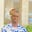Related Tags

javascript
communitycreator

# How to generate random whole numbers in JavaScriptAkande Olalekan Toheeb

### Overview

In this shot, we will learn to generate random numbers in JavaScript.

### The Math.random() and Math.floor()

In JavaScript, the Math.random() function generates random decimal numbers. The numbers generated are between 0, which is inclusive, and 1, which is exclusive. This means that the function can return 0 but not 1.

• JavaScript
Console

### Explanation

• Line 1: We create a function. This function prints a random number to the console. The number is between 0 and 1, where 1 is exclusive. We can refresh it to see the effect.
• Line 2: We call the function.

### Example

The Math. floor() function rounds down a number to its nearest whole number.

The code below prints 12 to the console:

Console

### How to generate whole numbers

To generate whole numbers, we combine the function of Math.random() with Math.floor(). This means we generate a random number, then round it down to its nearest whole number.

### Example

To generate a whole number between 0 and 24:

• We use the Math.random() function to generate a random decimal.
• We multiply the decimal by 25.
• We use the Math.floor() function to round down the number to its nearest whole number.

We can see this in the code below, which will print numbers between 0 and 24 to the console:

Console

We can generate random whole numbers that fall between two specific ranges of two numbers. A *minimum (min) and a maximum number ( max) are defined to get this.

We can use the formula below:


Math.floor(Math.random() * (max - min + 1) + min



Console

### Explanation

The code above returns a whole number between 1 and 50.

• Line 1: We create a function with two arguments, min and max. The formula for getting a random whole number within a range is passed to be printed in the console.

• Line 2: We call the function.

RELATED TAGS

javascript
communitycreator

CONTRIBUTORAkande Olalekan Toheeb
RELATED COURSES

View all Courses

Keep Exploring

Learn in-demand tech skills in half the time